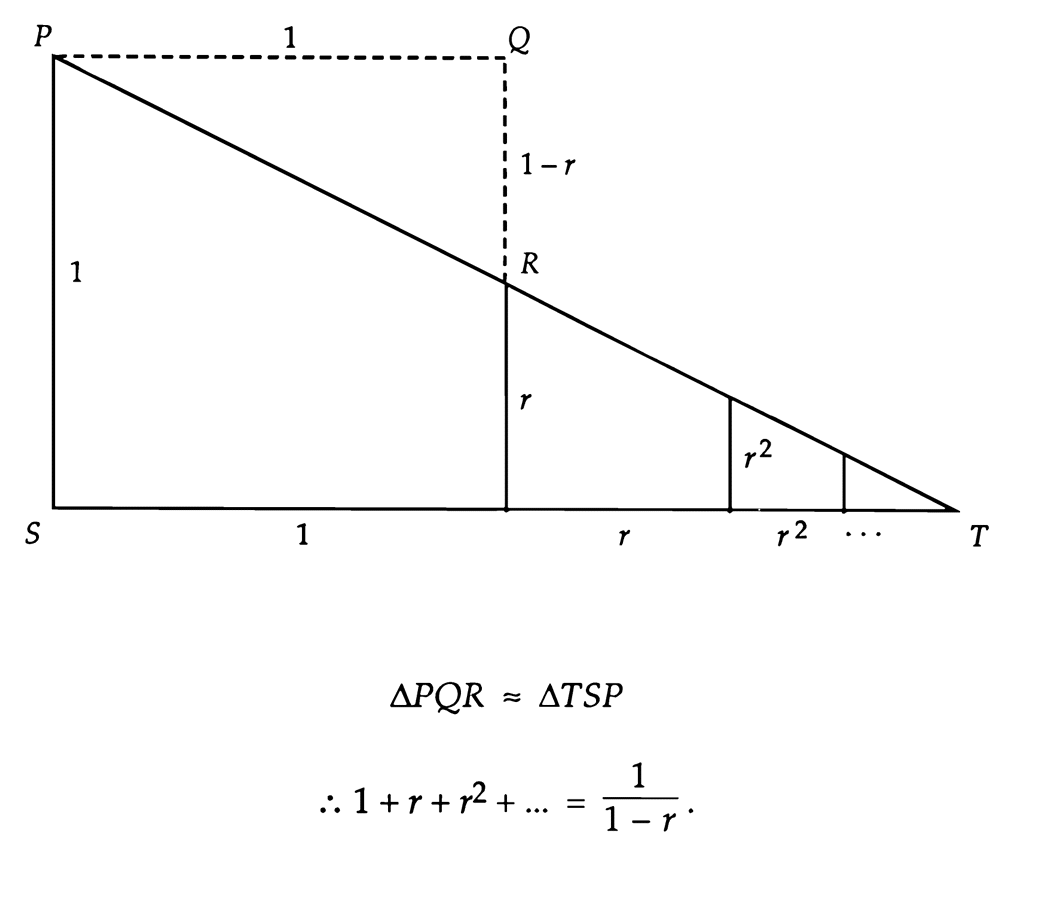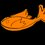A Visual Proof for Geometric SeriesI personally love the idea of Proofs Without Words! I like how simple, elegant and creative they can be. So here is another one for all you proof enthusiasts. Link

Expansion

In this proof, we see that $\frac{1}{1-r}$ = $1 + r + r^2 + r^3 ...$.

$\triangle TPS$ is cut into multiple similar triangles with a ratio r. ${ST}$ is $1 + r + r^2 + r^3 ...$. For the purposes of this proof, let the point where line ${QR}$ meets $ST$ be $A$.

$\angle PRQ = \angle TRA$ because they're vertical angles. $\angle PQR = \angle TAR$ since they are both right angles. Thus $\triangle PRQ \sim \triangle TRA$ by $AA$ Similarity. Since $\triangle TPS \sim \triangle TRA$, $\triangle PRQ \sim \triangle TPS$.

Tinkering with our ratios, we get $\frac{PQ}{QR} = \frac{TS}{SP}$

$\frac{1}{1-r} = \frac{1 + r + r^2 + r^3 ...}{1}$

Q.E.DNote by Sherry Sarkar
5 years, 9 months ago

This discussion board is a place to discuss our Daily Challenges and the math and science related to those challenges. Explanations are more than just a solution — they should explain the steps and thinking strategies that you used to obtain the solution. Comments should further the discussion of math and science.

When posting on Brilliant:

• Use the emojis to react to an explanation, whether you're congratulating a job well done , or just really confused .
• Ask specific questions about the challenge or the steps in somebody's explanation. Well-posed questions can add a lot to the discussion, but posting "I don't understand!" doesn't help anyone.
• Try to contribute something new to the discussion, whether it is an extension, generalization or other idea related to the challenge.
• Stay on topic — we're all here to learn more about math and science, not to hear about your favorite get-rich-quick scheme or current world events.

MarkdownAppears as
*italics* or _italics_ italics
**bold** or __bold__ bold

- bulleted
- list

• bulleted
• list

1. numbered
2. list

1. numbered
2. list
Note: you must add a full line of space before and after lists for them to show up correctly
paragraph 1

paragraph 2

paragraph 1

paragraph 2

> This is a quote
This is a quote
# I indented these lines
# 4 spaces, and now they show
# up as a code block.

print "hello world"
# I indented these lines
# 4 spaces, and now they show
# up as a code block.

print "hello world"
MathAppears as
Remember to wrap math in $$...$$ or $...$ to ensure proper formatting.
2 \times 3 $2 \times 3$
2^{34} $2^{34}$
a_{i-1} $a_{i-1}$
\frac{2}{3} $\frac{2}{3}$
\sqrt{2} $\sqrt{2}$
\sum_{i=1}^3 $\sum_{i=1}^3$
\sin \theta $\sin \theta$
\boxed{123} $\boxed{123}$

Sort by:

We will get the same answer if we extend the dotted triangle towards the right:

If we extend upto r^2, we get $(1+r)/(1- r^{2} )$ which can be simplified to get the same answer.

If we extend upto r^3, we get $(1+r+r^{2})/(1-r^{3})$ which again can be simplified to get the same result. And so on......

- 5 years, 7 months ago

Wow. Nice proof!

- 5 years, 9 months ago

that's fantastic!!!!!!!!!

- 5 years, 9 months ago

Elegant Maths... that's what i call it

- 5 years, 9 months ago

It's really amazing,

- 5 years, 9 months ago

Thanks! It's my first post, so I'm on the lines here. :D

- 5 years, 9 months ago

Nice one for the first post. Keep posting!

- 5 years, 9 months ago

This is awesome! Although perhaps it is worth noting that $|r| < 1$ for this to work. I wonder if I could think up a similar geometric proof for the more general formula: $1 + r + r^{2} +... = \dfrac{1-r^{n}}{1-r}$... Hmm...

- 5 years, 9 months ago

Thank you for the feedback, I forgot to add that detail!

- 5 years, 9 months ago